# Permutations And Combinations Simplified

Permutations and combinations are an essential part of statistics. They show up in a ton of different places when you are finding the probability of anything. But it can be hard to remember the exact formula for a permutation or a combination when you need it without looking it up.   This post will show you an easy, intuitive way to understand permutations and combinations so that you only need to remember one thing, and the rest you can just calculate when you need it.

## Permutations and Combinations Basics

Permutations and combinations are a way of determining how many different possibilities of something there are.

Permutations are what you use when the order matters. For instance, if 8 people are racing in a track meet, and you want to find the different ways they could get 1st, 2nd, and 3rd place, then the order matters. So you would use a permutation.

Combinations are what you use when the order doesn’t matter.   For instance, if you have 10 different pieces of clothing you want to take on a trip, but you can only fit 7 of them in your suitcase, it matters which 7 you pick, but it doesn’t matter what order you put them in the suitcase, so you would use a combination

## The Key Point

There is one key thing to know with Permutations and Combinations, and that is the Factorial.   Typically denoted with an exclamation point !

If you want to find how many different ways you can arrange 8 different items, it is 8 Factorial, which is 8 * 7 * 6 * 5 * 4 * 3 * 2 * 1.   What this represents is that when you make your first choice of items to arrange, you have 8 to choose from. When you make your second choice, there is one less so that you have 7 to choose from, then 6 and so on.

Everything with permutations and combinations are just different applications of the Factorial.

## Permutations – Slightly Simpler Than Combinations

Let’s go back to the track example. Let’s say that you have 8 people racing on the track. The total different orders they could come in are 8 !

8 * 7 * 6 * 5 * 4 * 3 * 2 * 1 = 40320

Now let’s say you only care about the order of the first three people on the track. Clearly we would have fewer than the full 8! different permutations, because we only care about how the first 3 people finished, not all 8. So in that case you have 8 options for first place, 7 options for 2nd place, and 6 options for 3rd place, and that’s it.

8 * 7 * 6 = 336

This is the permutations of 8, choosing 3.

Now there isn’t a function that lets us just multiply 8 * 7 * 6 easily.   If we wanted the order of everyone, then 8 Factorial lets us multiply 8, down through 1, but it doesn’t stop in the middle. The way we do this is by finding 8 Factorial, and then dividing by 5 factorial. We use 5! because there are 5 items left behind that we don’t care about (8-3 = 5) That ends up beingThe

( 5 * 4 * 3 * 2 * 1)

Cancels out of both the numerator and denominator, and we are left with 8 * 7 * 6.

So to find the permutations of a subset of a group, what we have just done is

• Find the permutations of the entire group ( 8! in this case)
• Divide by the permutations of the part of the group left behind ( 5! In this case)

Why are we dividing by the permutations of the parts left behind ?   Because we don’t care what order they are in, so we need to cancel out all different orderings that they can be in.

## This is a key point to remember

• If you want to find the permutations of something, use the factorial
• If you want to find the permutations of a subset, find the permutations of the entire group, and then divide by the permutations of the set left behind.

## Combinations – Build on Permutations

• Permutations – We take the factorial of the entire set to find out the number of possibilities
• Permutations with a subset – We take the factorial of the entire set, and then divide by the factorial of the left behind set

Well for combinations we still don’t care about the order of the left behind set, but we also don’t care about the order of the set that we have chosen.   So we start with the permutations of the entire set, then divide by the permutations of the left behind set, then divide by the permutations of the chosen set.

So if we have 10 different items of clothing, and we can only choose 7 to pack, so there are 3 left behind, the number of possibilities are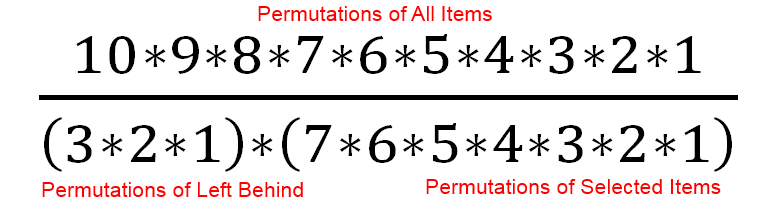Which is equal to 120

An important thing to know is that there will always be at least as many permutations of a set as combinations, and typically many more permutations than combinations.

## The Traditional Permutations & Combinations Equations

At this point, it is worth showing the traditional permutations and combinations equations. These are the things that you might typically be expected to memorize for a class, but can be challenging to remember long term

Here is the permutation equation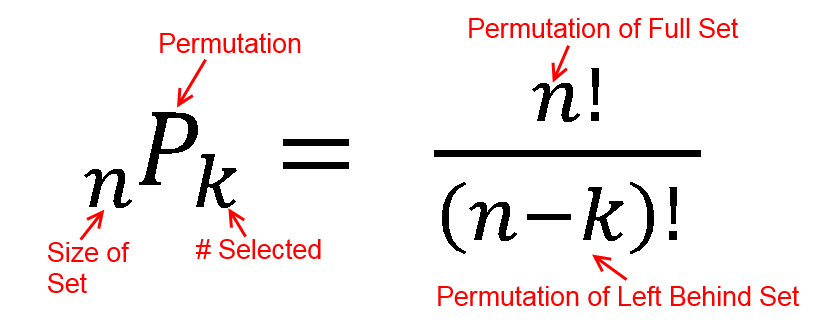And here is the combination equation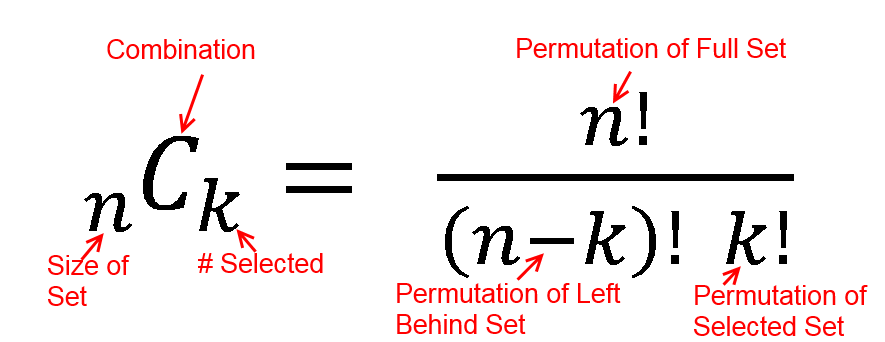So while it is manageable to memorize those equations, it is easier to just intuitively understand

• To find the permutations of a full set, take the factorial
• To find the permutations of a partial set, find the permutations of the full set, then divide by the permutations of the items left behind
• To find the combinations of a partial set, find the permutations of the full set, divide by the permutations of the items left behind, and divide by the permutations of the selected items.

## Taking It One Step Farther

If you understand using the factorial, instead of memorizing the equations, you can apply it to cases that the equations don’t cover. For example, if you have 12 items, and you need to break them up into 4 equally sized groups, how many options do you have ? (order does not matter within any group)

Once again you start with the permutations of the entire group, and then divide by the permutations of any subset whose order you don’t care about. In this case we have 4 subsets with 3 items each where the order doesn’t matter. So the equation would be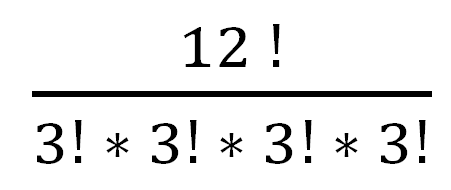Which is 19,958,400

That would be a challenging answer to get relying on just the standard equations

# Two Envelope Problem

The Two Envelope problem is one of my favorite statistical paradoxes. It is a problem that, even after you understand it, you can still revisit and be surprised by it.

## The Two Envelope Problem

You are presented with two identical envelopes. Both of the envelopes have money in them. You are told that one envelope has some amount of money in it, and the other envelope has twice as much money in it. But you don’t know how much that amount of money is. You are allowed to choose one envelope

You choose one envelope, which you decide to call Envelope A, and open it and observe X dollars. You are now given the opportunity to switch envelopes, so you calculate the expected value of switching. Since you know one envelope has twice as much money as the other, there is a 50% chance that envelope B, has twice as much as A, and a 50% chance that envelope B has half as much as A so

B = .5 * A/2 + .5 * 2A

B = 1.25 A

You conclude that the expected value of taking envelope B is 25% greater than keeping envelope A, so you start to switch. Before you do, you realize that if you had chosen envelope B to start with, you would have done the same calculation in reverse

A = .5 * B/2 + .5 * 2B

A = 1.25 B

and determined that the expected value of envelope A was 25% greater than envelope B.

How can you resolve this apparent paradox ?

## The Fairly Nerdy Solution

There are apparently quite a few solutions out there to this problem, with their authors believing their solution is clearly correct, and others disagreeing.   I’ll leave it to you to let me know what you think of my solution.

First we need to clarify how the money was put into the envelope, because it makes a big difference to the results.   The possible options are

• Coin Flip Scenario – The money is not put into the second envelope until you look at the first envelope.   i.e. if you see \$100 in the first envelope, then the game master flips a coin to decide whether to put \$200 or \$50 in the second envelope
• Infinite Money Scenario – The money is put into both of the envelopes before you choose. One envelope has X dollars and the other envelope has 2X dollars. The game master has infinite money, and would be willing to put any amount of money, including infinite money, into the envelopes
• Finite Money Scenario – The money is put into the both of the envelopes before you choose. One envelope has X dollars and the other envelope has 2X dollars.   However the game master is only willing to put a finite amount of money in the envelope, so there would be a maximum of M dollars in any envelope

Each of those options need to be considered separately, and I think that some confusion on this problem stems from not clearly defining those options

## Coin Flip Scenario

In my opinion, this scenario doesn’t really match the problem description of having two envelopes to choose from. However it does exactly match the math description of how the expected value of the second envelope is calculated.

If you are faced with this scenario, and see X dollars in your envelope, then your expected value of switching truly is 1.25 X, because the amount of money in envelope B can be calculated by

B = .5 * X/2 + .5 * 2X = 1.25X
which is how the problem is outlined.

This is basically a double or nothing bet, but instead of nothing, it’s double or half, and it is clearly a profitable bet to take.

## Infinite Money Scenario

To my mind, this is the least realistic scenario. Because while you could set up the coin flipping problem outlined above, or set up the finite money scenario outlined below, infinite money isn’t possible.   Even if you were playing the game with the richest person alive, or even the government of all of Earth, there is still some upper limit to the total amount of money. But if you do assume infinite money, what happens?

If you are assuming infinite money, the most reasonable assumption is that one envelope has some amount of money in it, between zero to infinity dollars, and the other has half that amount in it.

The first thing we notice is that the expected value of envelope A, taken all by itself, is infinity. This is because the average of all numbers between zero to infinity is infinity.   We then immediately observe that the expected value of envelope B is also infinity.   So after solving the equation of

B = .5 * A/2 + .5 * 2A

We are left with

B = 1.25 infinity

And

A = 1.25 infinity

So this scenario doesn’t really boil down to “why is the expected value of switching greater than the expected value of not switching”   it ends up being “Infinity has weird properties that don’t match our intuition”

## Finite Money Scenario

The scenario where the envelopes have finite money is the most interesting scenario in my opinion. Both because you could actually replicate it in real life, and because the math is interesting. With the finite money scenario, the most reasonable assumption for how it gets distributed is

• The game master puts a random amount of money in the first envelope, between zero dollars up to the maximum dollars he is willing to put in one envelope, denoted as M
• The game master puts half that amount of money in the second envelope
• The game master flips a fair coin to shuffle those envelopes so they are randomly presented as envelope A & envelope B

However, we are still posed with the problem, why does

B = .5 * A/2 + .5 * 2A = 1.25 A

and

A = .5 * B/2 + .5 * 2B = 1.25 B

The cause of this apparent paradox turns out to be bad intuition on the possible distribution of money in the envelopes. The assumption in this paradox is that the all dollar amounts are equally likely. Therefore you have a 50% chance of doubling your money, and a 50% chance of cutting it in half, just like in the coin flip scenario.

For that to be true, it implies a probability function that looks like this, where all numbers are equally likelyWhere M is the Maximum amount of money the game master would be willing to put in a single envelope. However the actual distribution of money in those envelopes will look like thisContrary to our assumptions, all dollar amounts are not equally likely.   There will actually be 3 times as many quantities below half of the maximum value as the number above half the max value.

So before going any farther, intuitively what will this mean ?   Well clearly the likelihood of doubling our money goes down if we have a larger amount of money.   So the equation of

B = 50% * A/2 + 50% * 2A

Doesn’t really apply if the chances of getting A/2 and 2A aren’t the same for every value of A.

Why are there more small numbers than big numbers in this distribution ?   Because of the way the money is distributed.   If we ran this many times, and randomly generated the money in the first envelope to be between 0 and M dollars over and over, and then sorted that money, we would end up with a distribution that looked like this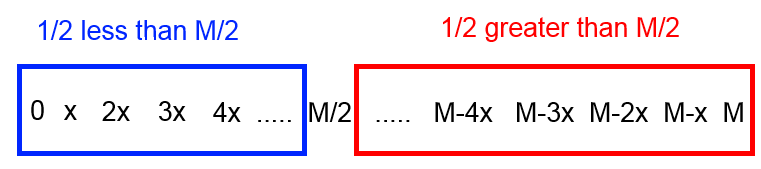Half of our numbers are less than M/2, and half are greater than M/2. Exactly what we intuitively expect.   When we generate the numbers in the second envelope by dividing all of these by 2 we end up with all of the numbers in the second envelope being less than M/2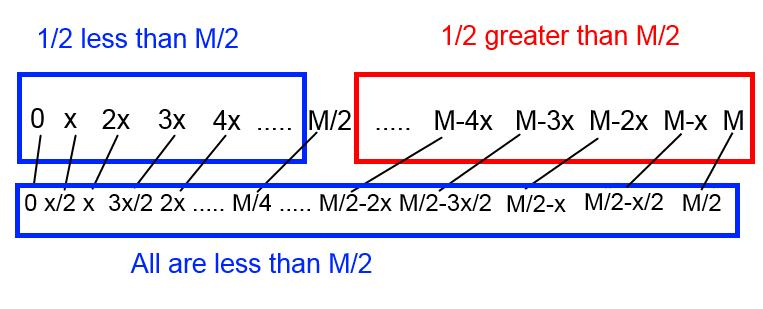In total, 1/4 of the numbers in both envelopes are greater than M/2, and 3/4 less than M/2.   Note, we set up these envelopes by putting up to a maximum amount into the first envelope and then dividing to get the second envelope. The 1/4 and 3/4 distribution would still hold if you went the other way and put up to a maximum amount into the first envelope and doubled it to get the second envelope.

At this point the intuitive solution to this paradox is clear. For any number there is not an even chance that we will double the value in the envelope vs the chance that we will cut that value in half.   The chance that we will double is larger for the small values than for the large values, which will mean that the

B = 1.25 A

A = 1.25B

Equations won’t hold.

The next section works out the math for exactly what the expected value of switching is for the finite money scenario. Then, in the final section we will exploit this information to see how if we were playing this game multiple times in a row, we actually could intelligently switch and end up with more money at the end of multiple rounds than the average expected value.

## Expected Value Of Switching – Finite Money Scenario

For calculating the expected value of switching, we will give a concrete example.   For this example, we will assume that the maximum amount of money that the game master is willing to put in an envelope is \$40.

In this scenario, the average value of the lower values is \$10, and the average value of the upper values is \$30.   The average value of all the money in the envelopes is \$15, calculated by .75 * \$10 + .25 * \$30 = \$15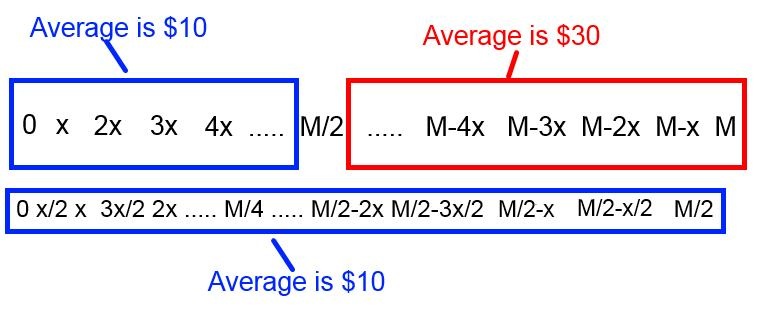At this point it’s easiest to assume that we will always get either the \$10 average value, or the \$30 average value when we open an envelope.   So when we open the first envelope, 75% of the time we will get \$10, and 25% of the time we will get \$30.So what is the expected value of switching if we have either the \$30 or the \$10 in the first envelope ?   For the \$30 it’s easy because if the first envelope has money that lies in the upper half of the range, switching will always get in the lower half of the range.   So the \$30 will always be cut in half to \$15

The \$10 is interesting because for every 3 times we see the \$10, 2 of those times we will double to \$20, and 1 time we will cut in half to \$5Breaking out the table for the expected value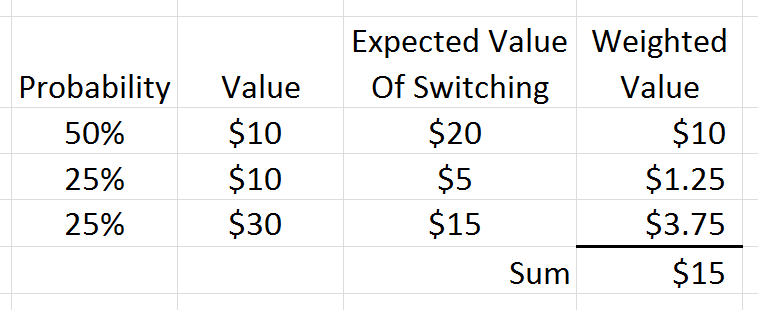Finding the weighted average of 50% of \$20, 25% of \$5, and 25% of \$15 gives us the total expected value of switching envelopes, which is \$15.

So the expected value of envelope A in this case is \$15, and if we switch, the expected value of envelope B is \$15, which are the same. This resolves our paradox, since we expect the average values in these envelopes to be the same

## Exploiting This Information

Interestingly, even though the average value of A & B are the same, this is still an exploitable game assuming:

• You play more than one round and
• You can look in your envelope before deciding whether you want to switch

Our earlier calculations show that the average value of all the switches was the same as not switching. But that was just the average value. Some of the individual switches themselves were beneficial, and some lost you money. If you can find a way to distinguish the profitable switches from the unprofitable ones, you can get more money.

The obvious solution is that you want to switch when you have the low dollar amount, and not when you have the high ones. The expected value of switching when you have the low dollar is:

• You will double your money 2 times out of 3
• You will lose half your money 1 time out of 3.On average switching when your envelope has less than half the maximum amount of money the game master would be willing to put in will yield +50% on those switches. Since you likely only have a rough guess as to how much money could be in the envelopes, a good (not necessarily perfect) strategy would be to switch envelopes the first time, and then after that switch if the amount in your envelope is less than half of the running maximum you have seen.

I simulated that strategy in the Excel below, playing 1000 games with a random maximum amount of money that could be in the envelope, random amounts in one envelope, half that amount in the other, randomly shuffled.   Whatever envelope got selected (from the random shuffle) we call envelope A, and the other envelope gets call B

Implementing that strategy gave approximately 25% more money than just picking the A or B envelopes.   You can download that Excel file here.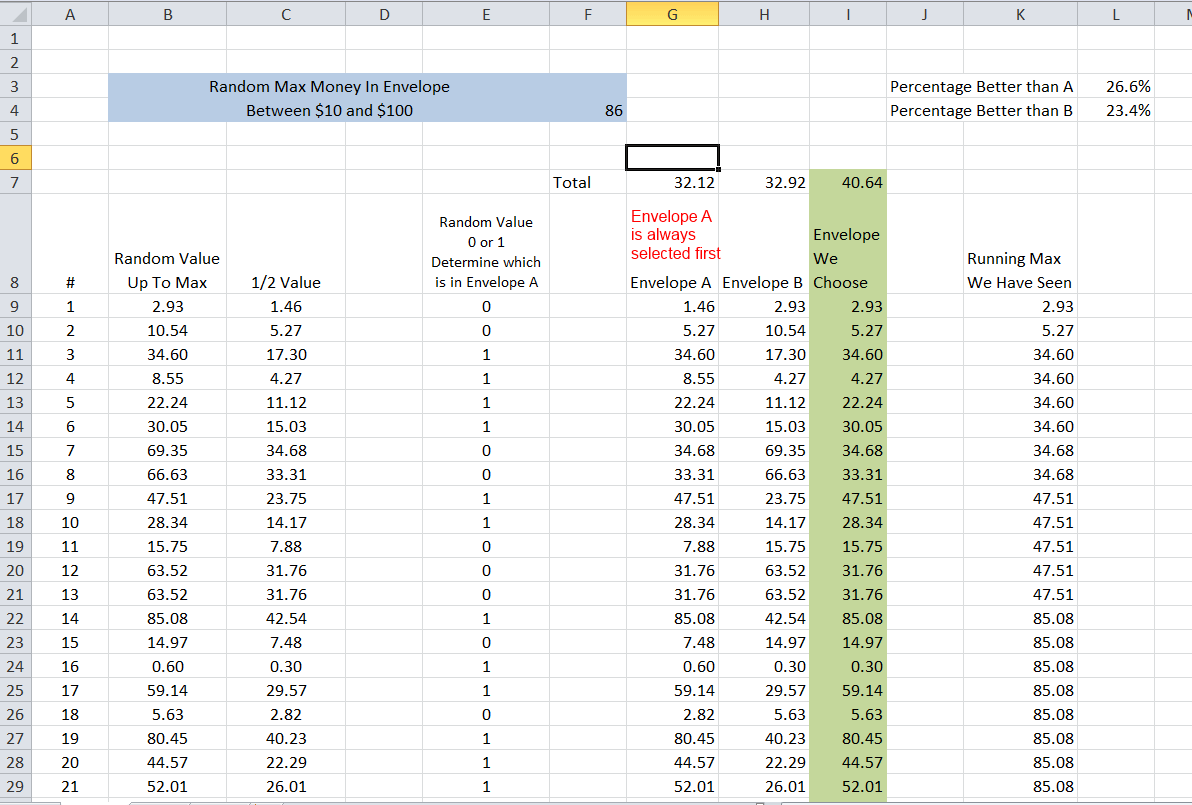# An Intuitive Guide To Bayes Theorem

The purpose of this page is to give you an intuitive understanding of how to solve Bayes Theorem problems.  The equation for Bayes Theorem is not all that clear, but Bayes Theorem itself is very intuitive.  The basics of Bayes Theorem are this

• Everything starts out with an initial probability – That is, before you do any tests or have any data, there is some initial probability of an event
• Tests can update that probability –  After you assign an initial probability, if you gather more information that is relevant then the probability can change.  For instance, you may initially have a very low chance of having an illness, but if a test for that illness comes back positive, the probability that you have it has increased
• After a test, all probabilities get normalized to 1 –  It doesn’t matter if an event is unlikely to have occurred.  What matters is if the event is likely compared to all other possible events.   For instance, if you don’t know whether you are observing a 6 sided die or a 20 sided die, and you see the die roll 4 five times in a row, it is unlikely that the 6 sided die would have rolled those values.  But it is extremely unlikely the 20 sided die would have, so comparatively the 6 sided die is more likely

## 6 Easy Steps For Any Bayes Problem

1. Determine what you want the probabilities for, and what you are observing
2. Estimate initial probabilities for all possible answers
3. For each of the initial possible answers, assume that it is true and calculate the probability of getting the observation with that possibility being true
4. Multiply the initial probabilities (Step 2) by the probabilities based on the observation (Step 3) for each of the initial possible answers
5. Normalize the results
6. Repeat steps 2-5 over and over for each new observation

## Bayes Theorem Applied To Cancer Testing

Testing for a disease is a classic Bayes Theorem problem, and one that can give counter intuitive results the first time you see it.   Let’s say that you are testing a generic patient for cancer.   One percent of the population has this cancer.   You have a test that will return a True Positive (return a positive when they actually do have cancer) 99% of the time, and return a True Negative (return a negative when they do not have cancer) 95% of the time.

You do 1 test, and get back a positive result.   What are the odds this patient actually does have this cancer ?

1. Determine Possibilities

There are two possibilities.  The patient either has cancer.  Or they do not have cancer

2. Estimate Initial Probabilities

Since  this is a generic patient they should be like the general population, so we assume there is a 1% chance they have cancer, and a 99% chance they do not.3. Calculate The Probability Of Getting The Result For Each Possible Answer

The result is a positive test.   If the patient has cancer, the probability of getting that result (True Positive) is 99%.   If the patient does not have cancer, the probability of getting that result (False Positive) is 5%  (which is 1 minus the 95% true negative rate)

4. Multiply Step 2 By Step 3 To Get The Combined Probability

This step should be similar to any other probability you have studied.  We are just calculating what is the probability they have cancer, and got a positive test.  And separately calculating what is the probability they do not have cancer, and got a positive test.5. Normalize The Results

This will be the final answer after 1 positive result.  At this step we see how likely the having cancer was, considering that a false positive was a possibilityAnd that is the answer, we have found that after the “99% Reliable” test, there is only a 16.7% chance that the patient has cancer

6. Repeat The Steps Over Again With Additional Observations

If you do additional tests, you use the new values as your starting probability.  In this case let’s assume that we do a second test, get a Positive result, and then a third test and get a Negative Result.

For the second test, the conditional equation is the same as the first test.  The normalized has cancer value of 16.7% gets multiplied by .99, and the normalized does not have cancer value of 83.33% gets multiplied by .05.

For the third test, since this was a negative result we need to change the formula.  We multiply the normalized has cancer probability by the False Negative rate of .01  (1-.99) , and the normalized does not have cancer rate by the True Negative rate of .95.

The results after both tests are shown below

##After the second positive result, the odds the patient actually has cancer jumps up to 79.8%, but after the negative test, the odds drop back down to 4%

## That Example Was Great, But You Promised Me Intuition

The page promised you intuition.  So far we have solved one Bayes Theorem problem, which is decent example, but not too different than what is on Wikipedia for Bayes Theorem.  Here is the intuition you should develop

• Bayes Theorem Is Just Multiplication and Division –  Bayes theorem itself is very simple.  Multiply out all of the strings of probabilities, and then normalize.  However some problems it is applied to are themselves very complicated, so the whole thing becomes complicated.  For instance, you can make the problem more difficult by using complicated probability distributions for the conditional probabilities or complicated initial probability distribution functions.  There might be special probability functions applied to a goal scoring problem in soccer, or a line waiting problem at a store.  But that doesn’t mean Bayes Theorem itself is all that complicated, the Bayes part of the problem is still just multiplication and division
• It is just as easy to solve for all possibilities as a single one – You might encounter a problem such as “This bag has 4 sided, 6 sided, 8 sided and 12 sided dice in it.  Your friend draws out a die, rolls it, and reports the number as 5.  What is the probability the selected die was a 6 sided die”  The problem asks you to solve for the 6 sided die, but since you have to get the total probability at each step any way in order to normalize, it is just as easy to solve the problem for the 4, 6, 8, and 12 sided dice at the same time.  Solving them all at the same time makes the thought process more straightforward, and can be done in a nice clean table.
• The order of observations doesn’t matter to end results – Bayes theorem amounts to repeated multiplication.  Multiplication is commutative.  You can change the order of the terms and get the same final results.   But if you change the order of the observations, for instance putting the negative cancer test result first in our example problem, the intermediate results will have different probabilities
• You Don’t Actually Have To Normalize Each Step – We normalized the example problem each step.  You could do all of the multiplication for the observations, and then normalize at the end and get the same result.  The only caution is that the probabilities can get very small after repeated decimal multiplication if you do not normalize. You can run into trouble with round off or truncation error depending on what you are using to do the math.

## So Why Was The Cancer Problem Result Surprising ?

Many people are surprised to see that a positive result on the 99% reliable test still only means there was a 16.7% chance the patient had cancer.  Why was that surprising ?  Because most people do not bake the initial probabilities into their intuition.

We do a good job of understanding the conditional probability.  After all, a 99% reliable test should make it much more likely the patient has cancer, which it does.  But if the initial probability is a really small number, the new probability will probably be small as well.  This often gets overlooked, and people implicitly assume an evenly distributed initial probability when thinking about these types of problems.

Overlooking the initial probability is the real joke behind this XKCD comic https://xkcd.com/1132/   (not having to pay the bet if the sun actually exploded is merely a bonus)

These pie charts are a good way to visualize what is occurring.  If the patient doesn’t do any test, the odds of having cancer are a small slice of the pie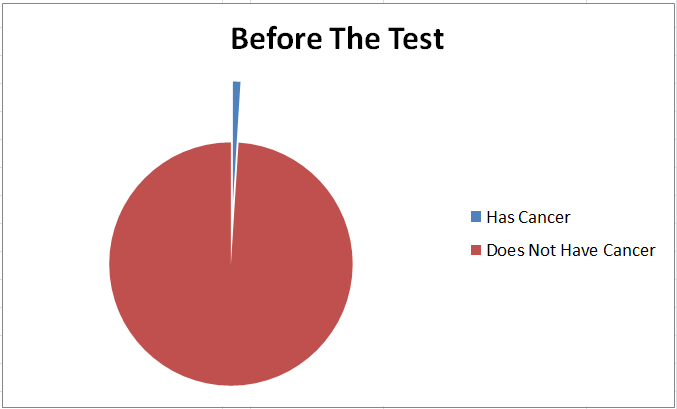While the patient is waiting for the test results there are 4 possibilities, either they have cancer and the test comes back positive (blue),  they don’t have cancer and the test comes back positive (green),  they don’t have cancer and the test comes back negative (purple)  or they have cancer and the test comes back negative (red slice, but too small to be seen)Once they get positive test results, the purple and red slices of the previous chart go away.   We normalize the green and blue slices in light of the new total probability. The odds of getting that result due to a false positive (green) are still larger than the odds of a true positive (blue).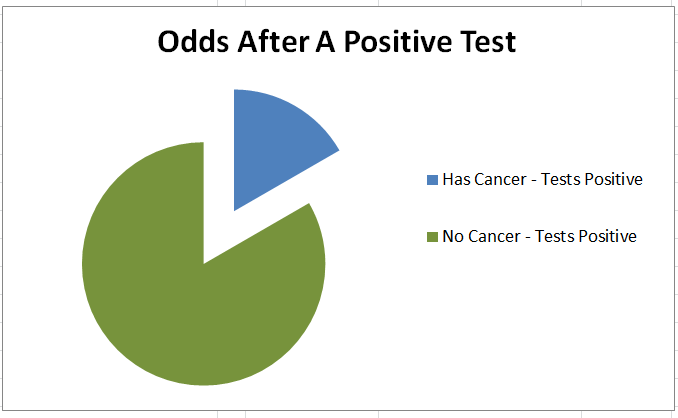## More Examples

If you want more examples and information about Bayes Theorem, here is a book I wrote walking through half a dozen Bayes Theorem examplesAnd here is an Excel file solution to some Bayes Theorem problems

# Understanding Statistical Significance

## Statistical Significance in Real Life

Statistical significance is a way of quantifying how unlikely something that you are measuring is, given what you know about the baseline.   Exactly how unlikely something needs to be before it is statistically significantly depends on the context.  You likely have an intuitive understanding of statistical significance based on your own life.

For instance,  if you were at a United States airport, and it was announced that your plane was 15 minutes late, you wouldn’t think that it was anything unusual.  But if you were at a Japanese bullet train station, and found out that it was going to be 15 minutes late, you would probably think that was at least somewhat odd.

Why does one seem like a more significant event than the other ?   It is because you know that planes are frequently late, where the trains almost never are.   So the trains being late is more significant because it is more different than the normal day to day variation than the plane being late.

## Plot The Delay

Statistical Significance is very easy to understand on a probability density plot.   The red line shows 15 minutes late.   The blue line shows how likely a train will be any given time late, and the green line shows how likely a plane will be any given time late.   The total area under each of the blue and green lines is 1

It is clear on the chart that very few trains are more than 15 minutes late, but  a lot of planes are.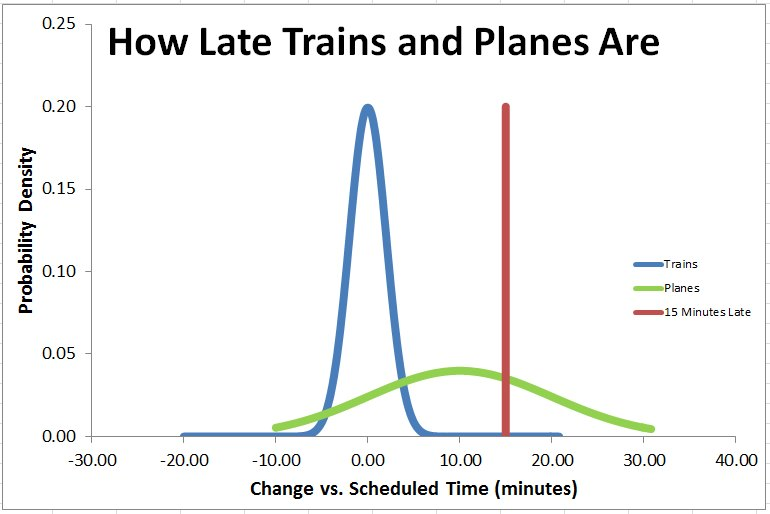There are really two things going on in the chart.  The first is that the average plane is more late than the average train is.   The average plane is 10 minutes late, and the average train is 0 minutes late.   So being 15 minutes late is bigger difference from average than for a train than a plane.

The second thing that is going on is that the distribution of plane lateness is a lot wider than the distribution of train lateness.  There is a lot more variation in the plane departure time than there is in the train departure time.   Because of that the plane lateness would have to be even greater to be unusual

## The Gist of Statistical Significance

Statistical Significance means quantifying the probability of how unlikely an event is.  Exactly what is statistically significant depends on context, but typical numbers considered statistically significant are if something would have less than a 5% chance, less than a 1% chance, or less than a .5% chance of occurring if there wasn’t some difference between what you are measuring and the baseline.

The information that is important to statistical significance are

• How many measurements you have – The more measurements you have, the more likely you have measured the full population of what is occurring, and not just a non-representative sample
• How different the average of your measurements is from the expected average  –  The bigger the difference, the more likely it is significant.
• How much variation there is in the measurements.  –   The less variation there is in the measurements, i.e. the tighter the spread is, the smaller the difference needs to be to be significant

There are small differences in the equations based on exactly what has been measured, but essentially all of the equations boil down to

• Get a number which is the difference in average values, multiplied by the square root of the number of measurements you have, and divided by the square root of the variation in your measures.   Call that number the “Test Statistic”
• The larger the Test Statistic the more statistically significant the difference.
• Look up the Test Statistic in the appropriate “Z-Table” or “T-Table” to find the probability that there is a statistically significant difference between your samples, as opposed to just random variation

## Equations For Statistical Significance

Now that you have a general understanding of Statistical Significance, it is time to look at the equations.   The most commonly used test for statistical significance is the Z-Test.   You use this test if you have a lot of measurements  (at least 20, preferably at least 40) and you are comparing it against a population with known values.   For example, you would use this test if you work at a hospital that had 500 babies born in it the past year, and you wanted to see if the average weight of those babies was different than the average weight of every baby born in your city.Where

• X_bar   : is the average of the measured data
• U_0      : is the population average
• Sigma  : is the population standard deviation
• n           : is the number of measured samples

You then look up the Z-value in a Z-Table to get probability

There are a few other different equations for Statistical significance called “T-Tests”.   You would use one of these T-Tests instead of a Z-test for one of these reasons

• The number of measurements you have is small, certainly you would use a T-Test with fewer than 20 measurements, or maybe fewer than 50
• You want to compare before and after measurements for the same individual.  For instance, if you have a before and after measurement for 20 people after a diet, you would use a certain type of T-Test.

## What is the difference between a Z-Test and a T-Test?

What is a T-test vs a Z-test, and how do you know when to use a Z-test or a T-test?  The thing to understand about T-Tests, is that they are almost the same as the Z-Test, and will give almost the same answer as you increase the number of measurements that you have.  The whole point of T-Tests is that they put more area at the tail of the normal curve, instead of the middle to account for uncertainty you would have in your measured mean and standard deviation if you have a very small sample size.   Once you get above 20 or so measurements the difference between Z-test results and T-test results becomes vanishingly small.

The plots below show the probability density for a Z-curve, and T-test curves with different sample sizesOnce you get past 20 or so measurements (green line, hardly visible) there really isn’t much of a difference between a T-Test or a Z-test (purple line).  However if you only have a few measurements than the T-Test results will need a lot greater Test Statistic to give a statistically significant result

It can be a little bit confusing knowing exactly which test to use, but using the exact right test isn’t that important unless you are taking an exam or righting a scientific paper.  The tests will all give similar results assuming you have more than 10 measurements, and very similar assuming you have 30 or more.

For a better understanding of the different types of tests, you can refer to this cheat sheet I put together giving the formulas for each test, and when they are used.## Examples of Z-Test vs T-Tests

This post was intending to give an intuitive understanding of statistical significance.   If you are interested in looking at examples of Z-Tests and T-tests and exactly how they are used and in what circumstance you might use one or the other, you can find some examples in this book I’ve put on AmazonOr you can get an Excel file with different hypothesis testing examples here.

# Bypassing WillpowerIf you want to accomplish something consistently, relying on willpower is the worst thing you can do.  As Jerry Seinfeld points out, your current self doesn’t really care about your future self.   If you want to work out consistently, you can’t rely on if you feel like working out.  Most likely how you are going to feel is too tired, or too busy.  Habits are one good way to bypass your willpower, but changing your environment is a better one.

Never Miss A Workout

Going for a run consistently is important to me.  But more often than not, once I get home from work I’m likely to not go out again.  The way around this is simple, Run Home.  Depending on where I’ve lived, I have had my wife drop me off at work, or taken the bus to work at the beginning of the day, and then changed clothes after work and run home.  When I live farther away from work, and take the bus, I have take the bus part of the way home, and get off on a stop that is 3-4 miles away, and run the rest of the way.  When I do this, I almost never miss a day of running.

The reason this works is that I have changed the value proposition.   Instead of getting home and having a choice between going running or relaxing, the choice is between running home or calling my wife and asking for a pickup.    What’s going to happen there is pretty clear.   I completely skip any opportunity for low willpower to matter.

But changing your environment isn’t just useful to make yourself exercise.   It is useful anytime your motivated planning self wants to make it impossible for your tired lazy self to wuss out or forget something.

For a while I’ve wanted to get ready for work faster in the morning. My previous habit was to wake up and eat breakfast while I use my computer, check email, surf, etc.    This always took a lot of time.  It was easy to do things slowly and waste time when I was groggy in the morning. I marveled at how quickly my wife was able to get ready in the morning.

But when I tried to resolve to either eat faster, or skip going on the computer, I always found myself back sliding.  I could be diligent for a few days, but not long term.   The problem was I was trying to rely on willpower at a time when my willpower was at its lowest.   Even waking up in the morning after getting little sleep due to the baby was a challenge.  Trying to force myself to operate at a higher level in that state was a fool’s game.

The solution was simple, change my environment.   I already routinely pack a lunch to take to work.   Now I also pack some toast and a hard boiled egg & maybe some yogurt to eat as breakfast right when I get to work.   It gives me an immediate energy boost to start the workday, and plugs a 20-30 minute leak in my morning routine.

The Practical Details

If you are going to manipulate your environment to bypass willpower, make sure you focus on the practical details.  For instance, when I run home from work, I want to carry as little in my backpack as possible.   Carrying shoes turned out to be a pain in the ass, so for a long time I just left my work shoes at my desk, and wore running shoes to and back from work.  Eventually I decided to embrace business casual, and just bought a pair of all black running shoes to wear and skipped the dress shoes entirely.

I’ve Never Regretted A Workout

Despite all this planning, there are still times I am forced to rely on willpower to work out at the end of the day.   When that happens, I try to remember   “I’ve never regretted a workout, but I’ve often regretted skipping one”

Photo at top of page from Flickr here

# Python Flash CardsI recently decided to buckle down and learn Python.   Python is a programming language that had been on my want list for some time.   It’s easy to use, has a ton of built in modules for things that I am interested in such as data science, and is also widely used at a lot of large tech companies.

After fiddling around with it for a while in 2014, I knew I liked the language, and could learn it, but I could never get over the hump.   You see, the problem was I was already really good at a different programming language.   I hired into a large aerospace company straight out of college, and being an old engineering company, FORTRAN was very ubiquitous.  Not to worry!   I had learned FORTRAN in high school  (along with Basic, Pascal, and C++) and while it was quite rusty after 5 years of little use, the value proposition was clear.   Dust off FORTRAN, and there was a ton of in house software that I could improve on to make my job easier.

As a result I became very good at FORTRAN, at least, very good for a mechanical engineer.  But 6 years later when I decided to update my skills and learn something new the value proposition was different.   Suddenly, for on the job work, the choice wasn’t   “Re-learn this coding language” vs.  “Do all this tedious work manually”  the choice was “Do the job in this new cool language, but one that will take a lot of Googling to get all the syntax”  vs.   “Get er done in the language I already know”

Since I didn’t use Python enough, I never got over the hump of “I need to Google 60-70% of what I need to do this job, and it will just take too long this time”

The solution was to take a page from my high school study techniques, and make Python Flash Cards.

So why did the flash cards help ?  Well for me the problem wasn’t understanding python syntax, I could read a program reasonably well, just not write it, nor was it understanding how to code since I was already fluent in one programming language and had previously known others well.  The problem was simply getting enough vocabulary down that I could do the simple jobs.

Once I had a foundation of vocabulary down, learning additional building blocks was fun and relatively easy.   Oh, should I use a dictionary here instead of a list?  That’s cool.   Is there a built in method of indexing my loop, so I don’t have to keep a separate counter!  What a great improvement to my code!    Once you know the basics, the great thing about learning programming is that since programmers write all of the software tools, and since they have made documentation one of the paragon virtues of their profession, there are just a ton of resources and learning communities out there.

Over the course of approximately a month, Python transitioned from a want to learn language for me, to one I was using for simple jobs, to one I was fluent in and use as my go to programming language.

I recommend using the flash cards in batches.   Instead of printing them all off and trying to learn them all at once, it is better to  work on 10 or 15 at a time and really hit them quite a few times in a short period.   I kept the flash cards at my desk and went through them when I got up to use the restroom or go to a meeting.   After you learn each batch, put it in the big stack of ones that you know, and review the big stack periodically.

Is Python something worth learning for you ?   Well if you already know at least one programming language, I’ll let you answer that for yourself.   But if you don’t know any, and you are someone who spends most of your work day on the computer, I posit that for you the answer is yes.   Simply being able to batch rename files alone saves me a lot of time, and if you work with a lot of text files or blocks of data the value proposition is even more clear.   Hopefully with these flash cards, the pain of starting will be low enough you can give it a go.

Let me know if they worked for you, and if you added improvements !

# 9 Baby Surprises

9 Biggest Surprises the First Year of Having A BabyMy wife and I thought that we were well prepared for having a baby.  We had read baby books, baby proofed the house, set up the crib and the diaper changing table, and above all, watched countless sitcoms where people have babies   (I’m looking at you  Rachel,  Phoebe, and Pam).   Here are the 9 biggest things that we didn’t know

1. Back Labor

Back labor was a surprise to us,  although apparently 25% of women experience it.   It was a Tuesday when I got home from work, and my wife said that she was experience back spasms.    I asked, do you think they are contractions ?    And she said she didn’t think so because she wasn’t feeling anything in her stomach.   So I asked her to tell me when the next couple were, and saw that they were spaced pretty evenly at about six minutes apart.     At that point we decided to call the doctor’s office and we got the nurse on call.

“Is this labor “   we asked,  or is it just Braxton Higgs contractions ?       “Well you will know that is is labor when your stomach gets hard like a basketball”    Was the reply.    So I asked my wife,   is your stomach getting hard like a basketball?     “No, it’s all in my back”.    So we waited a couple of hours, and the back spasms kept getting worse, so we decided to go in.

At the hospital, the nurse checked out my wife, and diagnosed her her as having back labor.   This was caused by the baby facing the wrong direction.   His head was facing forward, so the back of his was was pressed against my wife’s tailbone.

So my wife was in labor, but unfortunately was only 1cm dilated, so the hospital wouldn’t check us in.   “Can you give me something for the pain?” my wife asked ?    “Well sure” the nurse replied   “I can give you this muscle relaxant that will help the contractions that you are feeling”     “I’m having back labor though,  will it help me ?”     “Probably not”.      At this point the best advice they could give was to take a warm, relaxing bath.

We asked when we should come back, and the nurse said  “Normally we recommend coming in when your contractions are 3-5 minutes apart, but since yours are already 5 minutes apart, you should come in when they hurt so much you can’t walk or talk during a contraction”.    Which is clearly very unambiguous guidance.

1. Your Water May Not Break

So my wife was in labor, but not far enough along.    How long would it take to progress ?   Well according to the nurse, it could be anywhere from an hour to a week.    So we were premature going to the hospital, clearly we should have waited for my wife’s water to break.    We had a towel in the car, and that would be a definitive sign right ?    Wrong

We waited throughout the night and eventually decided we had to go back in.   We didn’t see any sign of my wife’s water breaking, but maybe we missed it.     We went in, and sure enough, my wife was at 4 cm and ready to be admitted to the hospital, but her water had not broken.  And in fact  never broke until the doctor assisted in that.     It turns out this was common too.   TV has lied to us

1. Check the hospital for a snack room

We were in the hospital for 3 days – 2 nights, and it wasn’t until an hour before checking out that I discovered there was a snack room for the mothers literally across the hall from the room we were in.   Up until that point my wife had been eating the hospital food, and food I got from the cafeteria, and asking the nurses to bring us the juice, or cheese or applesauce as a snack.    We didn’t realize it was all in a fridge just across the hall that we could help ourselves to without bothering the nurses.

1. No Sleep

Before having the baby, my friends and coworkers joked about   “Get your sleep in now”     but you really never know how serious people are.     After all, people complain about changing diapers, but we’ve never found them to be a problem.    The lack of sleep is real though.   My son is 13 months old now, and he literally has not slept a full night more than 5 nights so far.    Fortunately, at 13 months he’s getting up 1-2 times every night, which means we can actually survive.   For the first several months when he was getting up every 2 hours we were barely getting by

Sadly, even knowing this, there is not much to do about it.   If someone invents a way to store sleep for later, let me know and I will buy one.

1. Ear Infections Are Almost Impossible to Identify

In months 5-9 our son had ear infections 3-4 times, and he was put on amoxicillin and they cleared up nicely.   But despite the fact that we recognized all 4 ear infections for what they were, we probably had 8 or so false alarms.  The symptoms which we thought were sure fire indicators of the ear infection, such as ear tugging, crying when set on one side, waking up continuously through the night turned out to be very hit or miss.   So we had quite a few times taking a fussy baby to the doctors only to be told he was teething or there was nothing obviously the matter.

The doctors advised us that the ear infections were most likely to be associated with a cold, but we never really saw a cold any of the times, so really never got good at identifying the ear infections.

1. Both the baby and the mom need to learn how to nurse

I guess we expected that nursing would be instinctual.  But right from the beginning our son had trouble nursing.  With the help of the lactation consultant at the hospital, there were times when it went well, but we had a lot of trouble.  So much so, that after day 3 when our son had lost more than the recommended amount of birth weight  (lost 11%, greater than the 10% cutoff) the doctor recommended that we start using the finger & tube method for supplemental feeding.   I did that for a few weeks until we were able to get a working system with nursing and bottle feeding.

Although with the help of the lactation nurse at the hospital, our son was able to latch onto the bare nipple, we had a lot of trouble with that.  What eventually worked for us was using a nipple shield for the first 2-3 months, since that made it a lot easier for him to latch onto.   Eventually our son got the hang of it, and we stopped using the nipple shield since it was a hassle to wash it after every feeding.       –  Side Note –  If you use them, see if the hospital will give you a few spare ones before you go!

1. Kids can decide they hate to be spoon fed

Right around month 6-7, my son was eating a wide array of different baby food, and a very small assortment of cut up real food.   Well, quickly after he started eating the cut up real food, he decided that he was not interested in the baby food at all, and absolutely would not allow himself to be spoon fed by mommy or daddy.    And while he was happy enough to have the spoon himself, this did not result in eating so much as decorating the floors.

As a result, we fairly abruptly switched over to all sliced up real foods and ended up with a couple of weeks worth of cans of baby food that we had to give away.

1. Kids develop their favorite foods early

I’m not sure if my son had a favorite canned baby food, but as soon as we switched over to real food he had a clear favorite.  Blueberries.   Basically, he would eat most food pretty well, if he was hungry.  Some days he would eat a lot, some days not so much, but on any day he could eat basically unlimited numbers of blueberries.   We eventually starting making sure to wait until the end of the meal to get the blueberries out of the fridge, because once he saw them he would not eat anything else.

Nowadays, he definitely has some favorites, like plain, no sauce pasta, or sliced grapes, but no favorites that are quite as strong as blueberries

1. Some milestones are very fuzzy, others happen very quickly

When you hear your parents talk about first words you think one day you weren’t speaking, and the next you had a very definitive word.   With the baby, it’s clear that’s a much more fuzzy line.   Grandma & Grandpa have been quick to attribute many sounds to a first word.   ( Yes, he said “Da” when he saw me, but he says “Da” when he sees anyone, and babbles it all the time.  Can we count that ?)

For us, we settled on “Bir“ being his first word.   Sure it’s missing the “D” at the end, but he will repeatedly and consistently say it when looking at birds out the window, or pointing to birds in a book.

But as fuzzy as the talking milestone was, the walking milestone was crystal clear.   One day he was only cruising along the couch and tables, and the next he was taking a few steps, and within 3 days he was perfectly happy walking across the entire room.    This was surprising to me given how slow the crawling milestone was.   Around month 4-5 we had been consistently predicting that he would crawl any day now, and in fact he could move himself forward by month 3 provided you put your fists behind his feet to push off of.   But the crawling took a long time of slow progress until he was good at it, unlike the walking.

So that’s our top 9 surprises for the first year.   Undoubtedly he’ll find 9 or 99 new ones for year number 2.